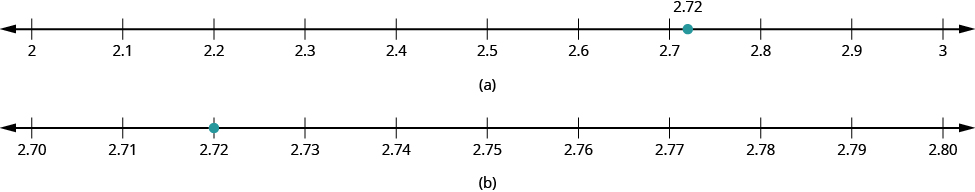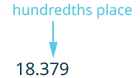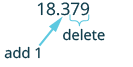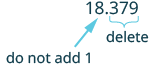## Rounding Decimals

### Learning Outcomes

• Round decimals

In the United States, gasoline prices are usually written with the decimal part as thousandths of a dollar. For example, a gas station might post the price of unleaded gas at $\3.279$ per gallon. But if you were to buy exactly one gallon of gas at this price, you would pay $3.28$ , because the final price would be rounded to the nearest cent. In a lesson about whole numbers, we learned that we can round numbers to get an approximate value when the exact value is not needed.

Suppose we wanted to round $2.72$ to the nearest dollar. Is it closer to $2$ or to $3$? What if we wanted to round $2.72$ to the nearest ten cents; is it closer to $2.70$ or to $2.80$? The number lines in the image below can help us answer those questions.

(a) We see that $2.72$ is closer to $3$ than to $2$. So, $2.72$ rounded to the nearest whole number is $3$.

(b) We see that $2.72$ is closer to $2.70$ than $2.80$. So we say that $2.72$ rounded to the nearest tenth is $2.7$.Can we round decimals without number lines? Yes! We use a method based on the one we used to round whole numbers.

### Round a decimal.

1. Locate the given place value and mark it with an arrow.
2. Underline the digit to the right of the given place value.
3. Is this digit greater than or equal to $5?$
• Yes – add $1$ to the digit in the given place value.
• No – do not change the digit in the given place value
4. Rewrite the number, removing all digits to the right of the given place value.

Tip:  If the digit to the right of your given place value is 5 or above, give your given place value a shove.  If the digit to the right of your given place value is 4 or less, let your given place value rest.

### example

Round $18.379$ to the nearest hundredth.

Solution

 $18.379$ Locate the hundredths place and mark it with an arrow.Underline the digit to the right of the $7$.Because $9$ is greater than or equal to $5$, add $1$ to the $7$.Rewrite the number, deleting all digits to the right of the hundredths place. $18.38$ $18.38$ is $18.379$ rounded to the nearest hundredth.

### example

Round $18.379$ to the nearest

1. tenth
2. whole number

Solution

 1. Round $18.379$ to the nearest tenth. $18.379$ Locate the tenths place and mark it with an arrow.Underline the digit to the right of the tenths digit.Because $7$ is greater than or equal to $5$, add $1$ to the $3$.Rewrite the number, deleting all digits to the right of the tenths place. $18.4$ So, $18.379$ rounded to the nearest tenth is $18.4$.
 2. Round $18.379$ to the nearest whole number. $18.379$ Locate the ones place and mark it with an arrow.Underline the digit to the right of the ones place.Since $3$ is not greater than or equal to $5$, do not add $1$ to the $8$.Rewrite the number, deleting all digits to the right of the ones place. $18$ So $18.379$ rounded to the nearest whole number is $18$.

### try it

Watch the following video to see an example of how to round a number to several different place values.

## Contribute!

Did you have an idea for improving this content? We’d love your input.